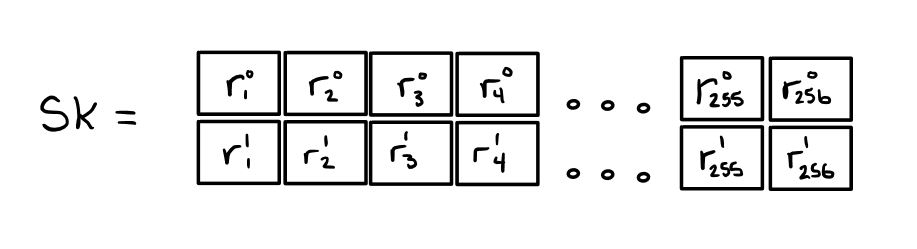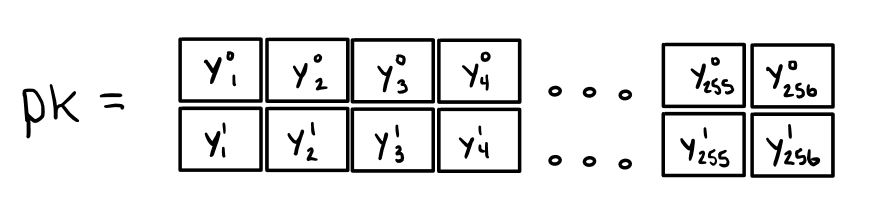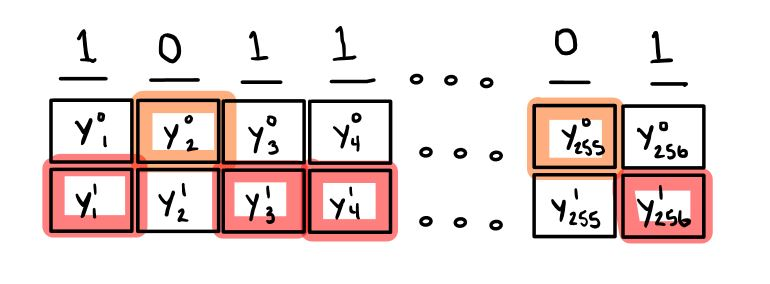# Implementing the Lamport one-time signature scheme in Python

Published:

Armed with a cryptographically secure one-way hash function and a secure source of randomness, we can build a digital signature scheme that is believed to be secure even with the advent of quantum computers. Created by Leslie Lamport at SRI International, the Lamport signature scheme is useful for many things.

In this post, I use SHA-256 as my cryptographically secure hash function. My implementation in Python uses the secrets library for generating random numbers (which defaults to using the most secure source of randomness provided by your operating system).

## Digital signatures

Suppose Alice wishes to broadcast messages to her many friends. She creates a message $m_{A} = \text{“Lamport signatures are cool! (from Alice)”}$ and broadcasts it. Her friend Bob receives this message and reads it. But how can Bob be sure that this message came from Alice, and is un-altered? For example, what if an evil person Eve writes a message $m_{E} = \text{“Lamport signatures are NOT cool! (from Alice)”}$ and broadcasts it? Bob will receive this message and think that Alice does not like Lamport signatures! Luckily for Alice, this is one of the many problems solved by digital signatures!

## Lamport Signatures

Lamport signatures are a one-time signature scheme that allows Alice to sign her messages. This signature allows Bob and others to verify that messages which say “(from Alice)” were indeed constructed by Alice.

### Key generation

Suppose Alice wishes to broadcast messages to her many friends. For every message, a new public key / secret key pair $(pk, sk)$ must be generated. The secret key $sk$ takes the form of a $256 \times 2$ matrix of random numbers $r_x$.From the secret key, another $256 \times 2$ matrix is created by hashing the secret key values: $y_{x}^{b} = SHA256(r_{x}^{b})$. This matrix is Alice’s public key $pk$.This public key $pk$ must then be distributed to message recipients who wish to verify the authenticity of Alice’s messages. For this post, we assume this is easy enough (spoiler alert: this is not easy).

All of Alice’s friends (and also Eve) have access to this public key $pk$ made available by Alice. However, only Alice has access to her secret key $sk$.

### Message signing

To sign the message $m_A = \text{“Lamport signatures are cool! (from Alice)”}$, Alice first hashes the message using a cryptographically secure hash function: $h_{m_A} = SHA256(m_A)$ and observes the binary representation of $h_{m_A}$. For each bit $b = \{0, 1\}$, Alice appends $sk_i^b$ to the message signature. For example, if $h_{m_A} = 1011…01$:The resulting message signature $s_{m_A}$ is therefore:Alice can then broadcast the message/signature pair $(m_A, s_{m_A})$ to all of her friends.

### Message verification

When Bob receives $(m_A, s_{m_A})$ over the air, he wants first to verify that it did indeed come from Alice. To do this, he uses his access to Alice’s public key $pk$. First, the message $m_A$ is hashed using the same cryptographically secure hash function that Alice used: $h_{m_A} = SHA256(m_A)$. For each bit $b$ in the binary representation of $h_{m_A}$, Bob appends the corresponding public key value $pk_i^b$ to a string of bytes.Finally, Bob can verify the signature $s_{m_A}$ by hashing each $256$-bit chunk of the signature, and verifying that it equals the corresponding string of bytes constructed from Alice’s public key:When Bob sees that these two values are equal, he can be sure that this message came from Alice. Furthermore, it is hard for some adversary (Eve) to forge signatures that Bob accepts.

## The hardness of forging a Lamport signature

Lamport signatures are secure due to the hardness of inverting a cryptographic hash function. Imagine Eve has the message $m_E = \text{“Lamport signatures are NOT cool! (from Alice)”}$, which she wishes to forge Alice’s signature for. For each bit $b$ in $h_{m_E} = SHA256(m_E)$, Eve must find a value $x_i$ such that $y_i^b = SHA256(x_i)$. This is difficult to do for many cryptographic hash functions (such as SHA-256), and is what is known as pre-image resistance. Furthermore, to forge signatures for arbitrary messages, Eve must do this for every $y^b_i$ i.e., find pre-images for $512$ different hashes provided by Alice’s public key. On the average case, a SHA-256 hash takes $2^{256}$ tries to find a pre-image. This is infeasbile and is what makes this signature scheme secure (as long as you only use each key once).

What happens if Alice uses a key to sign two different messages $m_1$ and $m_2$? Observe that $h_{m_1} = SHA256(m_1)$ and $h_{m_2} = SHA256(m_2)$, will differ in approximately half of their bits on average. For each bit $b_i$ where $h_{m_1}$ and $h_{m_2}$ differ, the adversary learns the secret key values $r_{i}^0$ and $r_{i}^1$ that are used to produce the corresponding values in the public key. For these bits, Eve is able to assign values arbitrarily.

As a toy example, consider the case where:

$h_{m_1} = 0100110…011$

$h_{m_2} = 1101011…010$

Eve will be able to construct messages of the form:

$h_{m_3} = SHA256(m_3) = b10bb1b…01b$

where each $b$ value can be either a $0$ or $1$. This implies that Alice loses about half of her security each time she re-uses her keypair to sign a message. Therefore, after re-using the same key to sign just $5$ messages, Alice reduces her security from a problem that would take over the age of the universe for Eve to solve in order to sign a single message, to allowing Eve to sign almost any message without having to solve anything.

## Key and signature size

Note that these digital signatures are not very succinct. The signature itself is $(256\cdot 256)$ bits $= 8$ kilobytes. Even worse, the public and private keys are each $2\cdot(256\cdot 256)$ bits $= 16$ kilobytes. This is not to say that signing, verification, and key generation are not fast or even that they are inferior to other digital signature schemes. However, in many settings this overhead in signature size can be an unacceptable cost e.g., power-constrained devices running on mobile ad hoc networks.

## Implementation in Python

Below is an implementation of the Lamport one-time signature scheme in Python3. I usually try to provide an interface for readers to try out the code from the browser (see my posts on Miller-Rabin primality test, solving discrete log in Bash, etc.); however, I’m not providing one for this post. The primary reason being that signatures are 8KB in size, and I don’t think that will display nicely on the page. Feel free to copy and paste the code and try it out on your own!

#!/usr/bin/python3

# Author: Zachary Ratliff
# Simple Python implementation of Lamport signature scheme

import hashlib
import math
import secrets
import sys

CHAR_ENC = 'utf-8'
BYTE_ORDER = sys.byteorder
SK = 0
PK = 1

# Generate a keypair for a one-time signature
def keygen():
sk = [[0 for x in range(256)] for y in range(2)]
pk = [[0 for x in range(256)] for y in range(2)]
for i in range(0,256):
#secret key
sk[i] = secrets.token_bytes(32)
sk[i] = secrets.token_bytes(32)
#public key
pk[i] = hashlib.sha256(sk[i]).digest()
pk[i] = hashlib.sha256(sk[i]).digest()

keypair = [sk,pk]
return keypair

# Sign messages using Lamport one-time signatures
def sign(m, sk):
sig = [0 for x in range(256)]
h = int.from_bytes(hashlib.sha256(m.encode(CHAR_ENC)).digest(), BYTE_ORDER)
for i in range(0,256):
b = h >> i & 1
sig[i] = sk[b][i]

return sig

# Verify Lamport message signatures
def verify(m, sig, pk):
h = int.from_bytes(hashlib.sha256(m.encode(CHAR_ENC)).digest(), BYTE_ORDER)
for i in range(0,256):
b = h >> i & 1
check = hashlib.sha256(sig[i]).digest()
if pk[b][i] != check:
return False

return True

keypair = keygen()
message = "Lamport signatures are cool!"
sig = sign(message, keypair[SK])
verify(message, sig, keypair[PK])



Tags: